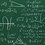# Muti-variable system of linear inequalties

We are all aware of the usual graphical method of solving a multi-variable system of inequalities. But how could one solve such a system algebraically? Also, does a system of inequalities follow the usual arithmetic of simultaneous equations? For instance, does the system $x+y>3 \\ x+2y>5$

necessarily imply $y>2$. ( It does not seem to, since $x=100$ and $y=1$ is a perfectly valid solution)4 years, 11 months ago

This discussion board is a place to discuss our Daily Challenges and the math and science related to those challenges. Explanations are more than just a solution — they should explain the steps and thinking strategies that you used to obtain the solution. Comments should further the discussion of math and science.

When posting on Brilliant:

• Use the emojis to react to an explanation, whether you're congratulating a job well done , or just really confused .
• Ask specific questions about the challenge or the steps in somebody's explanation. Well-posed questions can add a lot to the discussion, but posting "I don't understand!" doesn't help anyone.
• Try to contribute something new to the discussion, whether it is an extension, generalization or other idea related to the challenge.

MarkdownAppears as
*italics* or _italics_ italics
**bold** or __bold__ bold
- bulleted- list
• bulleted
• list
1. numbered2. list
1. numbered
2. list
Note: you must add a full line of space before and after lists for them to show up correctly
paragraph 1paragraph 2

paragraph 1

paragraph 2

[example link](https://brilliant.org)example link
> This is a quote
This is a quote
    # I indented these lines
# 4 spaces, and now they show
# up as a code block.

print "hello world"
# I indented these lines
# 4 spaces, and now they show
# up as a code block.

print "hello world"
MathAppears as
Remember to wrap math in $$ ... $$ or $ ... $ to ensure proper formatting.
2 \times 3 $2 \times 3$
2^{34} $2^{34}$
a_{i-1} $a_{i-1}$
\frac{2}{3} $\frac{2}{3}$
\sqrt{2} $\sqrt{2}$
\sum_{i=1}^3 $\sum_{i=1}^3$
\sin \theta $\sin \theta$
\boxed{123} $\boxed{123}$

Sort by:

No, algebra of inequalities is not that simple... You cannot just simply subtract or divide two inequalities like we do to two equations. As its evident that you have subtracted inequalitities which isn't allowed and hence gave wrong results..... As an explicit example, if it had been:

$x+y>3~~\text{and}~~ x+2y<5$ Then multiply first equation by $(-1)$ and add to second equation to get:

$-(\not x+y)+\not x+2y<2\iff y<2$

Note:- You can only add two inequalities​ together. You will not always be able to multiply, subtract or divide them.

PS:- I don't know any algebraic method to solve system these type of linear equations in two variables as I always relied on graph for getting solutions and they did the job fairly easily!

- 4 years, 11 months ago

Note: You cannot multiply two inequalities. For example, $-2 < 1$ and $-2 < 1$. Can we multiply them to conclude that $4 < 1$?

What is true is that you can multiply and divide by terms with the same sign. IE You can multiply/divide by $- (x+y)^2$, but not by $-x+y$.

Staff - 4 years, 11 months ago

True that.....

- 4 years, 11 months ago

Staff - 4 years, 11 months ago

But can we even subtract and divide two inequalities directly.. ??

- 4 years, 11 months ago

No, you cannot even multiply two inequalities. As stated,

What is true is that you can multiply and divide by terms with the same sign.

Note:- You can only add two inequalities​ together. You will not always be able to multiply, subtract or divide them

Staff - 4 years, 11 months ago

- 4 years, 11 months ago

- 4 years, 11 months ago

- 4 years, 11 months ago

- 4 years, 11 months ago

If I've understood what you mean I think you could divide this system in 3 disequalities (a>b>c --> a>b, b>c, a>c) but I don't know if it works. Anyway with the values x=100 and y=1 you obtain 101 > 302 > 5, that is not true.

- 4 years, 11 months ago

Sorry for the typo. I have edited the note

- 4 years, 11 months ago